x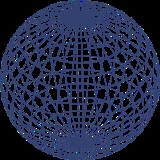SphereOverview

A sphere is a perfectly round geometrical object in three-dimensional space
Solid geometry
In mathematics, solid geometry was the traditional name for the geometry of three-dimensional Euclidean space — for practical purposes the kind of space we live in. It was developed following the development of plane geometry...

, such as the shape of a round ball
Ball
A ball is a round, usually spherical but sometimes ovoid, object with various uses. It is used in ball games, where the play of the game follows the state of the ball as it is hit, kicked or thrown by players. Balls can also be used for simpler activities, such as catch, marbles and juggling...

. Like a circle
Circle
A circle is a simple shape of Euclidean geometry consisting of those points in a plane that are a given distance from a given point, the centre. The distance between any of the points and the centre is called the radius....

in two dimensions, a perfect sphere is completely symmetrical around its center, with all points on the surface lying the same distance r from the center point. This distance r is known as the radius
Radius
In classical geometry, a radius of a circle or sphere is any line segment from its center to its perimeter. By extension, the radius of a circle or sphere is the length of any such segment, which is half the diameter. If the object does not have an obvious center, the term may refer to its...

of the sphere. The maximum straight distance through the sphere is known as the diameter
Diameter
In geometry, a diameter of a circle is any straight line segment that passes through the center of the circle and whose endpoints are on the circle. The diameters are the longest chords of the circle...

of the sphere.DiscussionsWhat are the two methods of development of a sphere?Encyclopedia
A sphere is a perfectly round geometrical object in three-dimensional space
Solid geometry
In mathematics, solid geometry was the traditional name for the geometry of three-dimensional Euclidean space — for practical purposes the kind of space we live in. It was developed following the development of plane geometry...

, such as the shape of a round ball
Ball
A ball is a round, usually spherical but sometimes ovoid, object with various uses. It is used in ball games, where the play of the game follows the state of the ball as it is hit, kicked or thrown by players. Balls can also be used for simpler activities, such as catch, marbles and juggling...

. Like a circle
Circle
A circle is a simple shape of Euclidean geometry consisting of those points in a plane that are a given distance from a given point, the centre. The distance between any of the points and the centre is called the radius....

in two dimensions, a perfect sphere is completely symmetrical around its center, with all points on the surface lying the same distance r from the center point. This distance r is known as the radius
Radius
In classical geometry, a radius of a circle or sphere is any line segment from its center to its perimeter. By extension, the radius of a circle or sphere is the length of any such segment, which is half the diameter. If the object does not have an obvious center, the term may refer to its...

of the sphere. The maximum straight distance through the sphere is known as the diameter
Diameter
In geometry, a diameter of a circle is any straight line segment that passes through the center of the circle and whose endpoints are on the circle. The diameters are the longest chords of the circle...

of the sphere. It passes through the center and is thus twice the radius.

In higher mathematics, a careful distinction is made between the sphere (a two-dimensional spherical surface embedded
Embedding
In mathematics, an embedding is one instance of some mathematical structure contained within another instance, such as a group that is a subgroup....

in three-dimensional Euclidean space
Euclidean space
In mathematics, Euclidean space is the Euclidean plane and three-dimensional space of Euclidean geometry, as well as the generalizations of these notions to higher dimensions...

) and the ball
Ball (mathematics)
In mathematics, a ball is the space inside a sphere. It may be a closed ball or an open ball ....

(the three-dimensional shape consisting of a sphere and its interior).

## Volume of a sphere

In 3 dimensions, the volume
Volume
Volume is the quantity of three-dimensional space enclosed by some closed boundary, for example, the space that a substance or shape occupies or contains....

inside a sphere (that is, the volume of a ball
Ball (mathematics)
In mathematics, a ball is the space inside a sphere. It may be a closed ball or an open ball ....

) is given by the formula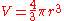where r is the radius of the sphere and π is the constant pi
Pi
' is a mathematical constant that is the ratio of any circle's circumference to its diameter. is approximately equal to 3.14. Many formulae in mathematics, science, and engineering involve , which makes it one of the most important mathematical constants...

. This formula was first derived by Archimedes
Archimedes
Archimedes of Syracuse was a Greek mathematician, physicist, engineer, inventor, and astronomer. Although few details of his life are known, he is regarded as one of the leading scientists in classical antiquity. Among his advances in physics are the foundations of hydrostatics, statics and an...

, who showed that the volume of a sphere is 2/3 that of a circumscribed cylinder
Cylinder (geometry)
A cylinder is one of the most basic curvilinear geometric shapes, the surface formed by the points at a fixed distance from a given line segment, the axis of the cylinder. The solid enclosed by this surface and by two planes perpendicular to the axis is also called a cylinder...

. (This assertion follows from Cavalieri's principle
Cavalieri's principle
In geometry, Cavalieri's principle, sometimes called the method of indivisibles, named after Bonaventura Cavalieri, is as follows:* 2-dimensional case: Suppose two regions in a plane are included between two parallel lines in that plane...

.) In modern mathematics, this formula can be derived using integral calculus, i.e. disk integration
Disk integration
Disk integration, , is a means of calculating the volume of a solid of revolution of a solid-state material, when integrating along the axis of revolution. This method models the generated 3 dimensional shape as a "stack" of an infinite number of disks of infinitesimal thickness...

to sum the volumes of an infinite number of circular disks of infinitesimal thickness stacked centered side by side along the x axis from x = 0 where the disk has radius r (i.e. y = r) to x = r where the disk has radius 0 (i.e. y = 0).

At any given x, the incremental volume (δV) is given by the product of the cross-sectional area of the disk at x and its thickness (δx):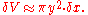The total volume is the summation of all incremental volumes: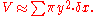In the limit as δx approaches zero this becomes: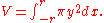At any given x, a right-angled triangle connects x, y and r to the origin, hence it follows from Pythagorean theorem
Pythagorean theorem
In mathematics, the Pythagorean theorem or Pythagoras' theorem is a relation in Euclidean geometry among the three sides of a right triangle...

that: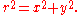Thus, substituting y with a function of x gives: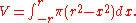This can now be evaluated:Therefore the volume of a sphere is: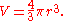Alternatively this formula is found using spherical coordinates, with volume element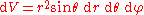In higher dimensions, the sphere (or hypersphere
Hypersphere
In mathematics, an n-sphere is a generalization of the surface of an ordinary sphere to arbitrary dimension. For any natural number n, an n-sphere of radius r is defined as the set of points in -dimensional Euclidean space which are at distance r from a central point, where the radius r may be any...

) is usually called an n-ball. General recursive formulas exist for deriving the volume of an n-ball
Deriving the volume of an n-ball
In geometry, the volume of a sphere is a special case of the n-dimensional volume of a ball in n-dimensional Euclidean space.-Derivation of the volume of an n-ball:...

.

For most practical uses, the volume of a sphere can be approximated as 52.4% of the volume of an inscribing cube, since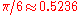. For example, since a cube with edge length 1 m has a volume of 1 m, a sphere with diameter 1 m has a volume of about 0.524 m.

## Surface area of a sphere

The surface area
Surface area
Surface area is the measure of how much exposed area a solid object has, expressed in square units. Mathematical description of the surface area is considerably more involved than the definition of arc length of a curve. For polyhedra the surface area is the sum of the areas of its faces...

of a sphere is given by the following formula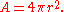This formula was first derived by Archimedes
Archimedes
Archimedes of Syracuse was a Greek mathematician, physicist, engineer, inventor, and astronomer. Although few details of his life are known, he is regarded as one of the leading scientists in classical antiquity. Among his advances in physics are the foundations of hydrostatics, statics and an...

, based upon the fact that the projection to the lateral surface of a circumscribing cylinder (i.e. the Lambert cylindrical equal-area projection
Lambert cylindrical equal-area projection
In cartography, the Lambert cylindrical equal-area projection, or Lambert cylindrical projection, is acylindrical, equal area map projection...

) is area-preserving. It is also the derivative
Derivative
In calculus, a branch of mathematics, the derivative is a measure of how a function changes as its input changes. Loosely speaking, a derivative can be thought of as how much one quantity is changing in response to changes in some other quantity; for example, the derivative of the position of a...

of the formula for the volume with respect to r because the total volume of a sphere of radius r can be thought of as the summation of the surface area of an infinite number of spherical shells of infinitesimal thickness concentrically stacked inside one another from radius 0 to radius r. At infinitesimal thickness the discrepancy between the inner and outer surface area of any given shell is infinitesimal and the elemental volume at radius r is simply the product of the surface area at radius r and the infinitesimal thickness.

At any given radius r, the incremental volume (δV) is given by the product of the surface area at radius r (A(r)) and the thickness of a shell (δr):The total volume is the summation of all shell volumes:In the limit as δr approaches zero this becomes: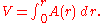Since we have already proved what the volume is, we can substitute V: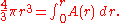Differentiating both sides of this equation with respect to r yields A as a function of r: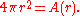Which is generally abbreviated as:Alternatively, the area element on the sphere is given in spherical coordinates by. With Cartesian coordinates, the area element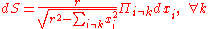. More generally, see area element.

The total area can thus be obtained by integration
Integral
Integration is an important concept in mathematics and, together with its inverse, differentiation, is one of the two main operations in calculus...

: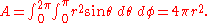## Equations in R3

In analytic geometry
Analytic geometry
Analytic geometry, or analytical geometry has two different meanings in mathematics. The modern and advanced meaning refers to the geometry of analytic varieties...

, a sphere with center (x0, y0, z0) and radius r is the locus
Locus (mathematics)
In geometry, a locus is a collection of points which share a property. For example a circle may be defined as the locus of points in a plane at a fixed distance from a given point....

of all points (x, y, z) such thatThe points on the sphere with radius r can be parametrized via(see also trigonometric function
Trigonometric function
In mathematics, the trigonometric functions are functions of an angle. They are used to relate the angles of a triangle to the lengths of the sides of a triangle...

s and spherical coordinates).

A sphere of any radius centered at zero is an integral surface of the following differential form
Differential form
In the mathematical fields of differential geometry and tensor calculus, differential forms are an approach to multivariable calculus that is independent of coordinates. Differential forms provide a better definition for integrands in calculus...

: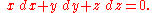This equation reflects the fact that the position and velocity vectors of a point travelling on the sphere are always orthogonal to each other.

The sphere has the smallest surface area among all surfaces enclosing a given volume and it encloses the largest volume among all closed surfaces with a given surface area. For this reason, the sphere appears in nature: for instance bubbles and small water drops are
roughly spherical, because the surface tension
Surface tension
Surface tension is a property of the surface of a liquid that allows it to resist an external force. It is revealed, for example, in floating of some objects on the surface of water, even though they are denser than water, and in the ability of some insects to run on the water surface...

locally minimizes surface area.
The surface area in relation to the mass of a sphere is called the specific surface area
Specific surface area
Specific surface area is a material property of solids which measures the total surface area per unit of mass, solid or bulk volume, or cross-sectional area...

. From the above stated equations it can be expressed as follows: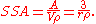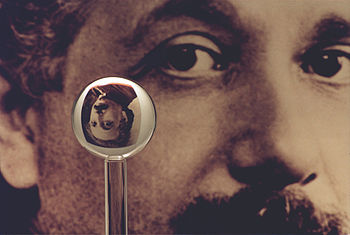A sphere can also be defined as the surface formed by rotating a circle
Circle
A circle is a simple shape of Euclidean geometry consisting of those points in a plane that are a given distance from a given point, the centre. The distance between any of the points and the centre is called the radius....

about any diameter
Diameter
In geometry, a diameter of a circle is any straight line segment that passes through the center of the circle and whose endpoints are on the circle. The diameters are the longest chords of the circle...

. If the circle is replaced by an ellipse
Ellipse
In geometry, an ellipse is a plane curve that results from the intersection of a cone by a plane in a way that produces a closed curve. Circles are special cases of ellipses, obtained when the cutting plane is orthogonal to the cone's axis...

, and rotated about the major axis, the shape becomes a prolate spheroid
Spheroid
A spheroid, or ellipsoid of revolution is a quadric surface obtained by rotating an ellipse about one of its principal axes; in other words, an ellipsoid with two equal semi-diameters....

, rotated about the minor axis, an oblate spheroid.

## Terminology

Pairs of points on a sphere that lie on a straight line through its center are called antipodal point
Antipodal point
In mathematics, the antipodal point of a point on the surface of a sphere is the point which is diametrically opposite to it — so situated that a line drawn from the one to the other passes through the centre of the sphere and forms a true diameter....

s.
A great circle
Great circle
A great circle, also known as a Riemannian circle, of a sphere is the intersection of the sphere and a plane which passes through the center point of the sphere, as opposed to a general circle of a sphere where the plane is not required to pass through the center...

is a circle on the sphere that has the same center and radius as the sphere, and consequently divides it into two equal parts. The shortest distance between two distinct non-antipodal points on the surface and measured along the surface, is on the unique great circle passing through the two points. Equipped with the great-circle distance
Great-circle distance
The great-circle distance or orthodromic distance is the shortest distance between any two points on the surface of a sphere measured along a path on the surface of the sphere . Because spherical geometry is rather different from ordinary Euclidean geometry, the equations for distance take on a...

, a great circle becomes the Riemannian circle
Riemannian circle
In metric space theory and Riemannian geometry, the Riemannian circle is a great circle equipped with its great-circle distance...

.

If a particular point on a sphere is (arbitrarily) designated as its north pole, then the corresponding antipodal point is called the south pole and the equator
Equator
An equator is the intersection of a sphere's surface with the plane perpendicular to the sphere's axis of rotation and containing the sphere's center of mass....

is the great circle that is equidistant to them. Great circles through the two poles are called lines (or meridians
Meridian (geography)
A meridian is an imaginary line on the Earth's surface from the North Pole to the South Pole that connects all locations along it with a given longitude. The position of a point along the meridian is given by its latitude. Each meridian is perpendicular to all circles of latitude...

) of longitude
Longitude
Longitude is a geographic coordinate that specifies the east-west position of a point on the Earth's surface. It is an angular measurement, usually expressed in degrees, minutes and seconds, and denoted by the Greek letter lambda ....

, and the line connecting the two poles is called the axis of rotation. Circles on the sphere that are parallel to the equator are lines of latitude
Latitude
In geography, the latitude of a location on the Earth is the angular distance of that location south or north of the Equator. The latitude is an angle, and is usually measured in degrees . The equator has a latitude of 0°, the North pole has a latitude of 90° north , and the South pole has a...

. This terminology is also used for astronomical bodies such as the planet Earth
Earth
Earth is the third planet from the Sun, and the densest and fifth-largest of the eight planets in the Solar System. It is also the largest of the Solar System's four terrestrial planets...

, even though it is not spherical and only approximately spheroid
Spheroid
A spheroid, or ellipsoid of revolution is a quadric surface obtained by rotating an ellipse about one of its principal axes; in other words, an ellipsoid with two equal semi-diameters....

al (see geoid
Geoid
The geoid is that equipotential surface which would coincide exactly with the mean ocean surface of the Earth, if the oceans were in equilibrium, at rest , and extended through the continents . According to C.F...

).

## Hemisphere

A sphere is divided into two equal hemispheres by any plane that passes through its center. If two intersecting planes pass through its center, then they will subdivide the sphere into four lunes
Lune (mathematics)
In geometry, a lune is either of two figures, both shaped roughly like a crescent Moon. The word "lune" derives from luna, the Latin word for Moon.-Plane geometry:...

or biangles, the vertices of which all coincide with the antipodal points lying on the line of intersection of the planes.

The antipodal quotient of the sphere is the surface called the real projective plane
Real projective plane
In mathematics, the real projective plane is an example of a compact non-orientable two-dimensional manifold, that is, a one-sided surface. It cannot be embedded in our usual three-dimensional space without intersecting itself...

, which can also be thought of as the northern hemisphere with antipodal points of the equator identified.

The round hemisphere is conjectured
Filling area conjecture
In mathematics, in Riemannian geometry, Mikhail Gromov's filling area conjecture asserts that among all possible fillings of the Riemannian circle of length 2π by a surface with the strongly isometric property, the round hemisphere has the least area...

to be the optimal (least area) filling of the Riemannian circle
Riemannian circle
In metric space theory and Riemannian geometry, the Riemannian circle is a great circle equipped with its great-circle distance...

.

If the planes don't pass through the sphere's center, then the intersection is called spheric section.

## Generalization to other dimensions

Spheres can be generalized to spaces of any dimension
Dimension
In physics and mathematics, the dimension of a space or object is informally defined as the minimum number of coordinates needed to specify any point within it. Thus a line has a dimension of one because only one coordinate is needed to specify a point on it...

. For any natural number
Natural number
In mathematics, the natural numbers are the ordinary whole numbers used for counting and ordering . These purposes are related to the linguistic notions of cardinal and ordinal numbers, respectively...

n, an n-sphere, often written as Sn, is the set of points in (n + 1)-dimensional Euclidean space which are at a fixed distance r from a central point of that space, where r is, as before, a positive real number. In particular:
• a 0-sphere is a pair of endpoints of an interval (−r, r) of the real line
• a 1-sphere is a circle
Circle
A circle is a simple shape of Euclidean geometry consisting of those points in a plane that are a given distance from a given point, the centre. The distance between any of the points and the centre is called the radius....

of radius r
• a 2-sphere is an ordinary sphere
• a 3-sphere
3-sphere
In mathematics, a 3-sphere is a higher-dimensional analogue of a sphere. It consists of the set of points equidistant from a fixed central point in 4-dimensional Euclidean space...

is a sphere in 4-dimensional Euclidean space.

Spheres for n > 2 are sometimes called hypersphere
Hypersphere
In mathematics, an n-sphere is a generalization of the surface of an ordinary sphere to arbitrary dimension. For any natural number n, an n-sphere of radius r is defined as the set of points in -dimensional Euclidean space which are at distance r from a central point, where the radius r may be any...

s.

The n-sphere of unit radius centred at the origin is denoted Sn and is often referred to as "the" n-sphere. Note that the ordinary sphere is a 2-sphere, because it is a 2-dimensional surface (which is embedded in 3-dimensional space).

The surface area of the (n − 1)-sphere of radius 1 is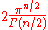where Γ(z) is Euler's Gamma function
Gamma function
In mathematics, the gamma function is an extension of the factorial function, with its argument shifted down by 1, to real and complex numbers...

.

Another formula for surface area is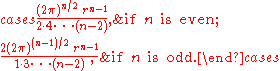and the volume is the surface area timesor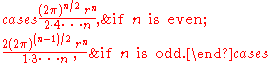## Generalization to metric spaces

More generally, in a metric space
Metric space
In mathematics, a metric space is a set where a notion of distance between elements of the set is defined.The metric space which most closely corresponds to our intuitive understanding of space is the 3-dimensional Euclidean space...

(E,d), the sphere of center x and radius is the set of points y such that d(x,y) = r.

If the center is a distinguished point considered as origin of E, as in a normed
Norm (mathematics)
In linear algebra, functional analysis and related areas of mathematics, a norm is a function that assigns a strictly positive length or size to all vectors in a vector space, other than the zero vector...

space, it is not mentioned in the definition and notation. The same applies for the radius if it is taken equal to one, as in the case of a unit sphere
Unit sphere
In mathematics, a unit sphere is the set of points of distance 1 from a fixed central point, where a generalized concept of distance may be used; a closed unit ball is the set of points of distance less than or equal to 1 from a fixed central point...

.

In contrast to a ball
Ball (mathematics)
In mathematics, a ball is the space inside a sphere. It may be a closed ball or an open ball ....

, a sphere may be an empty set, even for a large radius. For example, in Zn with Euclidean metric, a sphere of radius r is nonempty only if r2 can be written as sum of n squares of integers.

## Topology

In topology
Topology
Topology is a major area of mathematics concerned with properties that are preserved under continuous deformations of objects, such as deformations that involve stretching, but no tearing or gluing...

, an n-sphere is defined as a space homeomorphic to the boundary of an (n+1)-ball; thus, it is homeomorphic to the Euclidean n-sphere, but perhaps lacking its metric
Metric space
In mathematics, a metric space is a set where a notion of distance between elements of the set is defined.The metric space which most closely corresponds to our intuitive understanding of space is the 3-dimensional Euclidean space...

.
• a 0-sphere is a pair of points with the discrete topology
• a 1-sphere is a circle (up to
Up to
In mathematics, the phrase "up to x" means "disregarding a possible difference in  x".For instance, when calculating an indefinite integral, one could say that the solution is f "up to addition by a constant," meaning it differs from f, if at all, only by some constant.It indicates that...

homeomorphism
Homeomorphism
In the mathematical field of topology, a homeomorphism or topological isomorphism or bicontinuous function is a continuous function between topological spaces that has a continuous inverse function. Homeomorphisms are the isomorphisms in the category of topological spaces—that is, they are...

); thus, for example, (the image of) any knot
Knot (mathematics)
In mathematics, a knot is an embedding of a circle in 3-dimensional Euclidean space, R3, considered up to continuous deformations . A crucial difference between the standard mathematical and conventional notions of a knot is that mathematical knots are closed—there are no ends to tie or untie on a...

is a 1-sphere
• a 2-sphere is an ordinary sphere (up to
Up to
In mathematics, the phrase "up to x" means "disregarding a possible difference in  x".For instance, when calculating an indefinite integral, one could say that the solution is f "up to addition by a constant," meaning it differs from f, if at all, only by some constant.It indicates that...

homeomorphism
Homeomorphism
In the mathematical field of topology, a homeomorphism or topological isomorphism or bicontinuous function is a continuous function between topological spaces that has a continuous inverse function. Homeomorphisms are the isomorphisms in the category of topological spaces—that is, they are...

); thus, for example, any spheroid
Spheroid
A spheroid, or ellipsoid of revolution is a quadric surface obtained by rotating an ellipse about one of its principal axes; in other words, an ellipsoid with two equal semi-diameters....

is a 2-sphere

The n-sphere is denoted Sn. It is an example of a compact
Compact space
In mathematics, specifically general topology and metric topology, a compact space is an abstract mathematical space whose topology has the compactness property, which has many important implications not valid in general spaces...

topological manifold
Topological manifold
In mathematics, a topological manifold is a topological space which looks locally like Euclidean space in a sense defined below...

without boundary
Boundary (topology)
In topology and mathematics in general, the boundary of a subset S of a topological space X is the set of points which can be approached both from S and from the outside of S. More precisely, it is the set of points in the closure of S, not belonging to the interior of S. An element of the boundary...

. A sphere need not be smooth; if it is smooth, it need not be diffeomorphic to the Euclidean sphere.

The Heine-Borel theorem implies that a Euclidean n-sphere is compact. The sphere is the inverse image of a one-point set under the continuous function ||x||. Therefore, the sphere is closed. Sn is also bounded; therefore it is compact.

## Spherical geometry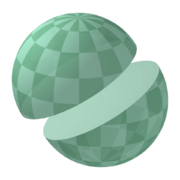The basic elements of plane geometry
Plane geometry
In mathematics, plane geometry may refer to:*Euclidean plane geometry, the geometry of plane figures,*geometry of a plane,or sometimes:*geometry of a projective plane, most commonly the real projective plane but possibly the complex projective plane, Fano plane or others;*geometry of the hyperbolic...

are points
Point (geometry)
In geometry, topology and related branches of mathematics a spatial point is a primitive notion upon which other concepts may be defined. In geometry, points are zero-dimensional; i.e., they do not have volume, area, length, or any other higher-dimensional analogue. In branches of mathematics...

and lines
Line (mathematics)
The notion of line or straight line was introduced by the ancient mathematicians to represent straight objects with negligible width and depth. Lines are an idealization of such objects...

. On the sphere, points are defined in the usual sense, but the analogue of "line" may not be immediately apparent. If one measures by arc length
Arc length
Determining the length of an irregular arc segment is also called rectification of a curve. Historically, many methods were used for specific curves...

one finds that the shortest path connecting two points lying entirely in the sphere is a segment of the great circle
Great circle
A great circle, also known as a Riemannian circle, of a sphere is the intersection of the sphere and a plane which passes through the center point of the sphere, as opposed to a general circle of a sphere where the plane is not required to pass through the center...

containing the points; see geodesic
Geodesic
In mathematics, a geodesic is a generalization of the notion of a "straight line" to "curved spaces". In the presence of a Riemannian metric, geodesics are defined to be the shortest path between points in the space...

. Many theorems from classical geometry hold true for this spherical geometry as well, but many do not (see parallel postulate
Parallel postulate
In geometry, the parallel postulate, also called Euclid's fifth postulate because it is the fifth postulate in Euclid's Elements, is a distinctive axiom in Euclidean geometry...

). In spherical trigonometry
Spherical trigonometry
Spherical trigonometry is a branch of spherical geometry which deals with polygons on the sphere and the relationships between the sides and the angles...

, angle
Angle
In geometry, an angle is the figure formed by two rays sharing a common endpoint, called the vertex of the angle.Angles are usually presumed to be in a Euclidean plane with the circle taken for standard with regard to direction. In fact, an angle is frequently viewed as a measure of an circular arc...

s are defined between great circles. Thus spherical trigonometry is different from ordinary trigonometry
Trigonometry
Trigonometry is a branch of mathematics that studies triangles and the relationships between their sides and the angles between these sides. Trigonometry defines the trigonometric functions, which describe those relationships and have applicability to cyclical phenomena, such as waves...

in many respects. For example, the sum of the interior angles of a spherical triangle exceeds 180 degrees. Also, any two similar spherical triangles are congruent.

## Eleven properties of the sphere

In their book Geometry and the imagination David Hilbert
David Hilbert
David Hilbert was a German mathematician. He is recognized as one of the most influential and universal mathematicians of the 19th and early 20th centuries. Hilbert discovered and developed a broad range of fundamental ideas in many areas, including invariant theory and the axiomatization of...

and Stephan Cohn-Vossen
Stephan Cohn-Vossen
Stefan or Stephan Cohn-Vossen was a mathematician, now best known for his collaboration with David Hilbert on the 1932 book Anschauliche Geometrie, translated into English as Geometry and the Imagination. The Cohn-Vossen transformation is also named for him.He was born in Breslau...

describe eleven properties of the sphere and discuss whether these properties uniquely determine the sphere. Several properties hold for the plane
Plane (mathematics)
In mathematics, a plane is a flat, two-dimensional surface. A plane is the two dimensional analogue of a point , a line and a space...

which can be thought of as a sphere with infinite radius. These properties are:
1. The points on the sphere are all the same distance from a fixed point. Also, the ratio of the distance of its points from two fixed points is constant.
The first part is the usual definition of the sphere and determines it uniquely. The second part can be easily deduced and follows a similar result of Apollonius of Perga
Apollonius of Perga
Apollonius of Perga [Pergaeus] was a Greek geometer and astronomer noted for his writings on conic sections. His innovative methodology and terminology, especially in the field of conics, influenced many later scholars including Ptolemy, Francesco Maurolico, Isaac Newton, and René Descartes...

for the circle
Circle
A circle is a simple shape of Euclidean geometry consisting of those points in a plane that are a given distance from a given point, the centre. The distance between any of the points and the centre is called the radius....

. This second part also holds for the plane
Plane (mathematics)
In mathematics, a plane is a flat, two-dimensional surface. A plane is the two dimensional analogue of a point , a line and a space...

.
2. The contours and plane sections of the sphere are circles.
This property defines the sphere uniquely.
3. The sphere has constant width and constant girth.
The width of a surface is the distance between pairs of parallel tangent planes. There are numerous other closed convex surfaces which have constant width, for example the Meissner body. The girth of a surface is the circumference of the boundary of its orthogonal projection on to a plane. It can be proved that each of these properties implies the other.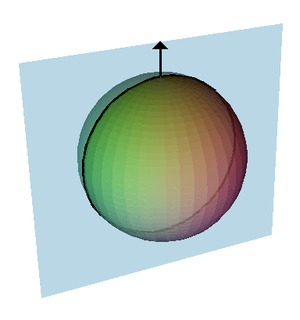4. All points of a sphere are umbilics.
At any point on a surface we can find a normal direction
Surface normal
A surface normal, or simply normal, to a flat surface is a vector that is perpendicular to that surface. A normal to a non-flat surface at a point P on the surface is a vector perpendicular to the tangent plane to that surface at P. The word "normal" is also used as an adjective: a line normal to a...

which is at right angles to the surface, for the sphere these are the lines radiating out from the center of the sphere. The intersection of a plane containing the normal with the surface will form a curve called a normal section and the curvature of this curve is the sectional curvature. For most points on most surfaces, different sections will have different curvatures; the maximum and minimum values of these are called the principal curvature
Principal curvature
In differential geometry, the two principal curvatures at a given point of a surface are the eigenvalues of the shape operator at the point. They measure how the surface bends by different amounts in different directions at that point.-Discussion:...

s. It can be proved that any closed surface will have at least four points called umbilical point
Umbilical point
In the differential geometry of surfaces in three dimensions, umbilics or umbilical points are points that are locally spherical. At such points both principal curvatures are equal, and every tangent vector is a principal direction....

s
. At an umbilic all the sectional curvatures are equal; in particular the principal curvature
Principal curvature
In differential geometry, the two principal curvatures at a given point of a surface are the eigenvalues of the shape operator at the point. They measure how the surface bends by different amounts in different directions at that point.-Discussion:...

s are equal. Umbilical points can be thought of as the points where the surface is closely approximated by a sphere.
For the sphere the curvatures of all normal sections are equal, so every point is an umbilic. The sphere and plane are the only surfaces with this property.
5. The sphere does not have a surface of centers.
For a given normal section there is a circle whose curvature is the same as the sectional curvature, is tangent to the surface and whose center lines along on the normal line. Take the two centers corresponding to the maximum and minimum sectional curvatures: these are called the focal points, and the set of all such centers forms the focal surface
Focal surface
For a surface in three dimension the focal surface, surface of centers or evolute is formed by taking the centers of the curvature spheres, which are the tangential spheres whose radii are the reciprocals of one of the principal curvatures at the point of tangency...

.
For most surfaces the focal surface forms two sheets each of which is a surface and which come together at umbilical points. There are a number of special cases. For channel surface
Channel surface
A channel or canal surface is a surface formed as the envelope of a family of spheres whose centers lie on a space curve. One sheet of the focal surface of a channel surface will be the generating curve....

s one sheet forms a curve and the other sheet is a surface; For cones
Cone (geometry)
A cone is an n-dimensional geometric shape that tapers smoothly from a base to a point called the apex or vertex. Formally, it is the solid figure formed by the locus of all straight line segments that join the apex to the base...

, cylinders, torus
Torus
In geometry, a torus is a surface of revolution generated by revolving a circle in three dimensional space about an axis coplanar with the circle...

es and cyclides both sheets form curves. For the sphere the center of every osculating circle is at the center of the sphere and the focal surface forms a single point. This is a unique property of the sphere.
6. All geodesics of the sphere are closed curves.
Geodesics are curves on a surface which give the shortest distance between two points. They are a generalization of the concept of a straight line in the plane. For the sphere the geodesics are great circles. There are many other surfaces with this property.
7. Of all the solids having a given volume, the sphere is the one with the smallest surface area; of all solids having a given surface area, the sphere is the one having the greatest volume.
These properties define the sphere uniquely. These properties can be seen by observing soap bubble
Soap bubble
A soap bubble is a thin film of soapy water enclosing air, that forms a hollow sphere with an iridescent surface. Soap bubbles usually last for only a few seconds before bursting, either on their own or on contact with another object. They are often used for children's enjoyment, but they are also...

s. A soap bubble will enclose a fixed volume and due to surface tension
Surface tension
Surface tension is a property of the surface of a liquid that allows it to resist an external force. It is revealed, for example, in floating of some objects on the surface of water, even though they are denser than water, and in the ability of some insects to run on the water surface...

its surface area is minimal for that volume. This is why a free floating soap bubble approximates a sphere (though external forces such as gravity will distort the bubble's shape slightly).
8. The sphere has the smallest total mean curvature among all convex solids with a given surface area.
The mean curvature
Mean curvature
In mathematics, the mean curvature H of a surface S is an extrinsic measure of curvature that comes from differential geometry and that locally describes the curvature of an embedded surface in some ambient space such as Euclidean space....

is the average of the two principal curvatures and as these are constant at all points of the sphere then so is the mean curvature.
9. The sphere has constant positive mean curvature.
The sphere is the only imbedded surface without boundary or singularities with constant positive mean curvature. There are other immersed surfaces with constant mean curvature. The minimal surface
Minimal surface
In mathematics, a minimal surface is a surface with a mean curvature of zero.These include, but are not limited to, surfaces of minimum area subject to various constraints....

s have zero mean curvature.
10. The sphere has constant positive Gaussian curvature.
Gaussian curvature
Gaussian curvature
In differential geometry, the Gaussian curvature or Gauss curvature of a point on a surface is the product of the principal curvatures, κ1 and κ2, of the given point. It is an intrinsic measure of curvature, i.e., its value depends only on how distances are measured on the surface, not on the way...

is the product of the two principal curvatures. It is an intrinsic property which can be determined by measuring length and angles and does not depend on the way the surface is embedded
Embedding
In mathematics, an embedding is one instance of some mathematical structure contained within another instance, such as a group that is a subgroup....

in space. Hence, bending a surface will not alter the Gaussian curvature and other surfaces with constant positive Gaussian curvature can be obtained by cutting a small slit in the sphere and bending it. All these other surfaces would have boundaries and the sphere is the only surface without boundary with constant positive Gaussian curvature. The pseudosphere
Pseudosphere
In geometry, the term pseudosphere is used to describe various surfaces with constant negative gaussian curvature. Depending on context, it can refer to either a theoretical surface of constant negative curvature, to a tractricoid, or to a hyperboloid....

is an example of a surface with constant negative Gaussian curvature.
11. The sphere is transformed into itself by a three-parameter family of rigid motions.
Consider a unit sphere placed at the origin, a rotation around the x, y or z axis will map the sphere onto itself, indeed any rotation about a line through the origin can be expressed as a combination of rotations around the three coordinate axis, see Euler angles
Euler angles
The Euler angles are three angles introduced by Leonhard Euler to describe the orientation of a rigid body. To describe such an orientation in 3-dimensional Euclidean space three parameters are required...

. Thus there is a three-parameter family of rotations which transform the sphere onto itself, this is the rotation group
Rotation group
In mechanics and geometry, the rotation group is the group of all rotations about the origin of three-dimensional Euclidean space R3 under the operation of composition. By definition, a rotation about the origin is a linear transformation that preserves length of vectors and preserves orientation ...

, SO(3). The plane is the only other surface with a three-parameter family of transformations (translations along the x and y axis and rotations around the origin). Circular cylinders are the only surfaces with two-parameter families of rigid motions and the surfaces of revolution
Surface of revolution
A surface of revolution is a surface in Euclidean space created by rotating a curve around a straight line in its plane ....

and helicoid
Helicoid
The helicoid, after the plane and the catenoid, is the third minimal surface to be known. It was first discovered by Jean Baptiste Meusnier in 1776. Its name derives from its similarity to the helix: for every point on the helicoid there is a helix contained in the helicoid which passes through...

s are the only surfaces with a one-parameter family.

## Cubes in relation to spheres

For every sphere there are multiple cuboids that may be inscribed within the sphere. When briefly considered it becomes apparent that the largest of the multiple cuboids which may be inscribed is a cube
Cube
In geometry, a cube is a three-dimensional solid object bounded by six square faces, facets or sides, with three meeting at each vertex. The cube can also be called a regular hexahedron and is one of the five Platonic solids. It is a special kind of square prism, of rectangular parallelepiped and...

.

## See also

• 3-sphere
3-sphere
In mathematics, a 3-sphere is a higher-dimensional analogue of a sphere. It consists of the set of points equidistant from a fixed central point in 4-dimensional Euclidean space...

• Affine sphere
Affine sphere
In mathematics, and especially differential geometry, an affine sphere is a hypersurface for which the affine normals all intersect in a single point...

• Alexander horned sphere
Alexander horned sphere
The Alexander horned sphere is a wild embedding of a sphere into space, discovered by . It is the particular embedding of a sphere in 3-dimensional Euclidean space obtained by the following construction, starting with a standard torus:...

• Ball (mathematics)
Ball (mathematics)
In mathematics, a ball is the space inside a sphere. It may be a closed ball or an open ball ....

• Banach–Tarski paradox
Banach–Tarski paradox
The Banach–Tarski paradox is a theorem in set theoretic geometry which states the following: Given a solid ball in 3-dimensional space, there exists a decomposition of the ball into a finite number of non-overlapping pieces , which can then be put back together in a different way to yield two...

• Cube
Cube
In geometry, a cube is a three-dimensional solid object bounded by six square faces, facets or sides, with three meeting at each vertex. The cube can also be called a regular hexahedron and is one of the five Platonic solids. It is a special kind of square prism, of rectangular parallelepiped and...

• Cuboid
Cuboid
In geometry, a cuboid is a solid figure bounded by six faces, forming a convex polyhedron. There are two competing definitions of a cuboid in mathematical literature...

• Curvature
Curvature
In mathematics, curvature refers to any of a number of loosely related concepts in different areas of geometry. Intuitively, curvature is the amount by which a geometric object deviates from being flat, or straight in the case of a line, but this is defined in different ways depending on the context...

• Directional statistics
• Dome (mathematics)
Dome (mathematics)
In mathematics, a dome is a closed geometrical surface which can be obtained by sectioning off a portion of a sphere with an intersecting plane. It consists of two parts: a flat disk, which is joined to a convex surface whose curvature is uniform and which has a circular boundary: this boundary...

• Dyson sphere
Dyson sphere
A Dyson sphere is a hypothetical megastructure originally described by Freeman Dyson. Such a "sphere" would be a system of orbiting solar power satellites meant to completely encompass a star and capture most or all of its energy output...

• Hoberman sphere
Hoberman sphere
A Hoberman sphere is a structure invented by Chuck Hoberman that resembles a geodesic dome, but is capable of folding down to a fraction of its normal size by the scissor-like action of its joints...

• Homology sphere
Homology sphere
In algebraic topology, a homology sphere is an n-manifold X having the homology groups of an n-sphere, for some integer n ≥ 1. That is,andTherefore X is a connected space, with one non-zero higher Betti number: bn...

• Homotopy groups of spheres
Homotopy groups of spheres
In the mathematical field of algebraic topology, the homotopy groups of spheres describe how spheres of various dimensions can wrap around each other. They are examples of topological invariants, which reflect, in algebraic terms, the structure of spheres viewed as topological spaces, forgetting...

• Homotopy sphere
Homotopy sphere
In algebraic topology, a branch of mathematics, a homotopy sphere is an n-manifold homotopy equivalent to the n-sphere. It thus has the same homotopy groups and the same homology groups, as the n-sphere...

• Hypersphere
Hypersphere
In mathematics, an n-sphere is a generalization of the surface of an ordinary sphere to arbitrary dimension. For any natural number n, an n-sphere of radius r is defined as the set of points in -dimensional Euclidean space which are at distance r from a central point, where the radius r may be any...

• Metric space
Metric space
In mathematics, a metric space is a set where a notion of distance between elements of the set is defined.The metric space which most closely corresponds to our intuitive understanding of space is the 3-dimensional Euclidean space...

• Napkin ring problem
Napkin ring problem
In geometry, it is somewhat surprising that the volume of a band of specified height around a sphere—the part that remains after a hole in the shape of a circular cylinder is drilled through the sphere—does not depend on the sphere's radius....

• Pseudosphere
Pseudosphere
In geometry, the term pseudosphere is used to describe various surfaces with constant negative gaussian curvature. Depending on context, it can refer to either a theoretical surface of constant negative curvature, to a tractricoid, or to a hyperboloid....

• Riemann sphere
Riemann sphere
In mathematics, the Riemann sphere , named after the 19th century mathematician Bernhard Riemann, is the sphere obtained from the complex plane by adding a point at infinity...

• Smale's paradox
Smale's paradox
In differential topology, Smale's paradox states that it is possible to turn a sphere inside out in a three-dimensional space with possible self-intersections but without creating any crease, a process often called sphere eversion...

• Solid angle
Solid angle
The solid angle, Ω, is the two-dimensional angle in three-dimensional space that an object subtends at a point. It is a measure of how large that object appears to an observer looking from that point...

• Sphere packing
Sphere packing
In geometry, a sphere packing is an arrangement of non-overlapping spheres within a containing space. The spheres considered are usually all of identical size, and the space is usually three-dimensional Euclidean space...

• Spherical cap
Spherical cap
In geometry, a spherical cap is a portion of a sphere cut off by a plane. If the plane passes through the center of the sphere, so that the height of the cap is equal to the radius of the sphere, the spherical cap is called a hemisphere....

• Spherical helix
• Spherical sector
Spherical sector
In geometry, a spherical sector is a portion of a sphere defined by a conical boundary with apex at the center of the sphere. It can be described as the union of a spherical cap and the cone formed by the center of the sphere and the base of the cap....

• Spherical segment
Spherical segment
In geometry, a spherical segment is the solid defined by cutting a sphere with a pair of parallel planes.It can be thought of as a spherical cap with the top truncated, and so it corresponds to a spherical frustum...

• Spherical shell
Spherical shell
In geometry, a spherical shell is a generalization of an annulus to three dimensions. A spherical shell is the region between two concentric spheres of differing radii....

• Spherical wedge
Spherical wedge
In geometry, a spherical wedge or ungula is a portion of a ball bounded by two plane semidisks and a spherical lune . The angle between the radii lying within the bounding semidisks is the dihedral angle of the wedge α...

• Spherical zone
• Spherical coordinates
• Spherical Earth
Spherical Earth
The concept of a spherical Earth dates back to ancient Greek philosophy from around the 6th century BC, but remained a matter of philosophical speculation until the 3rd century BC when Hellenistic astronomy established the spherical shape of the earth as a physical given...

• Zoll sphere
Zoll surface
In mathematics, a Zoll surface, named after Otto Zoll, is a surface homeomorphic to the 2-sphere , equipped with a Riemannian metric all of whose geodesics are closed and of equal length. While the usual unit-sphere metric on S2 obviously has this property, it also has an infinite-dimensional...

## External links

The source of this article is wikipedia, the free encyclopedia.  The text of this article is licensed under the GFDL.